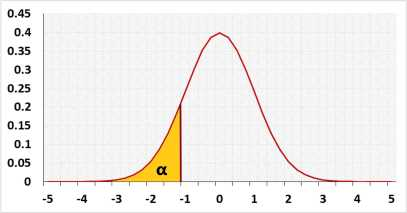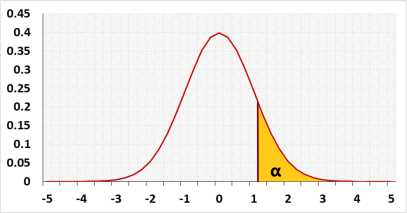# Power analysis calculator

## Distribution## Power calculation

Calculate the test power basted on the sample size and draw a power analysis chart.

Use this test for one of the following tests:
One Sample Z-Test
One Sample T-Test
Two Sample Z-Test
Two Sample T-Test, Equal σ (Pooled variance)
Two Sample T-Test, Unequal σ (Welch's)

### Statistic Data

 digits: 12345676910 example digits=2: (0.001234 => 0.0012) tails: two (Ho:μ=μ)left (Ho:μ≥μ)right (Ho:μ≤μ) distribution: normalt sample: one sampletwo samples standard deviations: equal σunequal σ α: significant level (0-1), maximum chance allowed rejecting H0 while H0 is correct (Type1 Error) effect size (unstandardized):standardized effect size: The power is expected to identify the effect. If one exists, H0 will be rejected. effect size (unstandardized) - the change the test is expected to identifystandardized effect size - the change divided by the standard deviation. examples:0.2 - small effect, 0.5 - medium , 0.8 - large σ1: standard deviation σ2: standard deviation n1: Group1 sample size n2: Group1 sample size

validation message